Histogram of Oriented Gradients(HOG), one of the well-known image processing algorithms, is a feature descriptor that is used for extracting essential features and shapes of a particular object within an image such as edges and textures. Features extracted by HOG can be used to feed into machine learning and deep learning model. The paper, “Histograms of Oriented Gradients for Human Detection”, was written by two French researchers: Navneet Dalal and Bill Triggs, has been widespread and popular since 2005 although the concept behind HOG was implemented early with a patent application in 1986.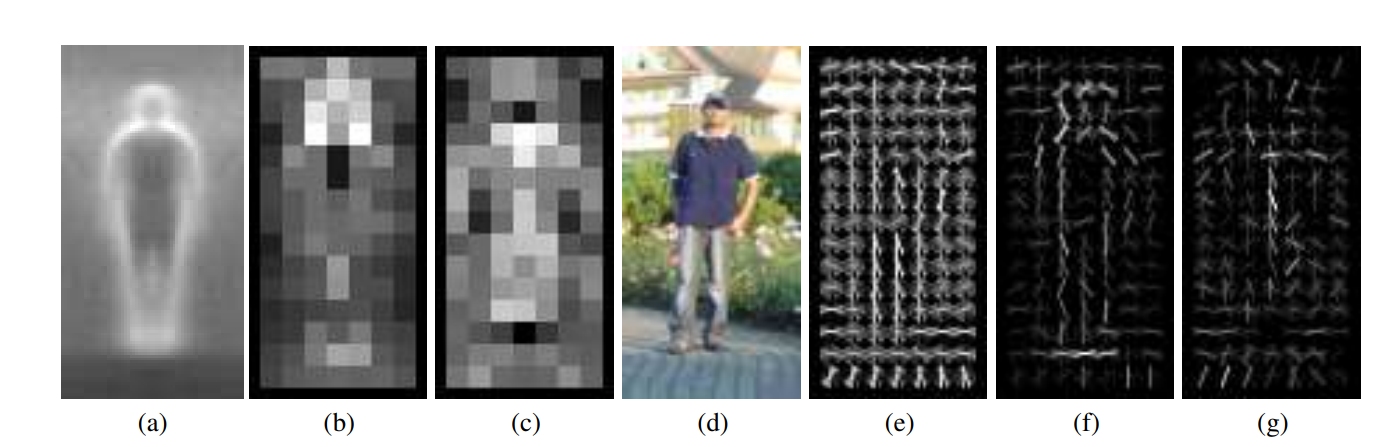Figure (1) - Pedestrian detection with HOG taken from Histograms of Oriented Gradients for Human Detection

HOG converts an image having (width x height x dimension) into a feature vector, which spatial dimension is relatively smaller than the original image. Therefore, HOG can be used to detect small-scaled images with less computational power, which means you can run HOG without having a powerful GPU.
One of the drawbacks of HOG is that its computation speed is tardy while detecting an object for large-scaled images as it uses a sliding window technique to extract features from every pixel of an image. Hence, the accuracy is not highly reliable compared to the current convolutional neural networks. However, HOG is still advantageous for objection detection in the computer vision industry because of its computation speed and accuracy. HOG is widely used for the detection of pedestrians and medical image analysis.

The role of feature descriptor is used to define an image by its color intensities of pixels. The feature descriptor tries to capture all edges or curves within an image in order to know outline information. Using the first order directive kernels, you can calculate gradient magnitude and then obtain the orientation. Each orientation is assigned to different bins to obtain a histogram. In each bin, gradient magnitudes are stored according to their orientation angles.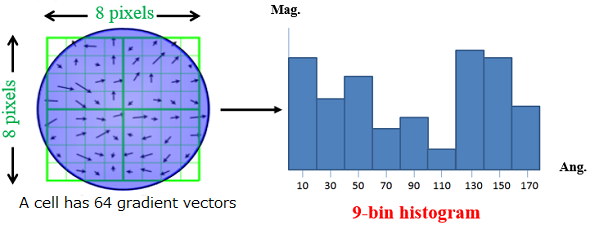Figure (3) - Histogram for gradient magnitude and orientation [Picture taken from 20th LSI Design Contests in Okinawa Design Specification — 3–1]

We will delve more into HOG algorithms to see how it works by approaching steps by steps without using a module.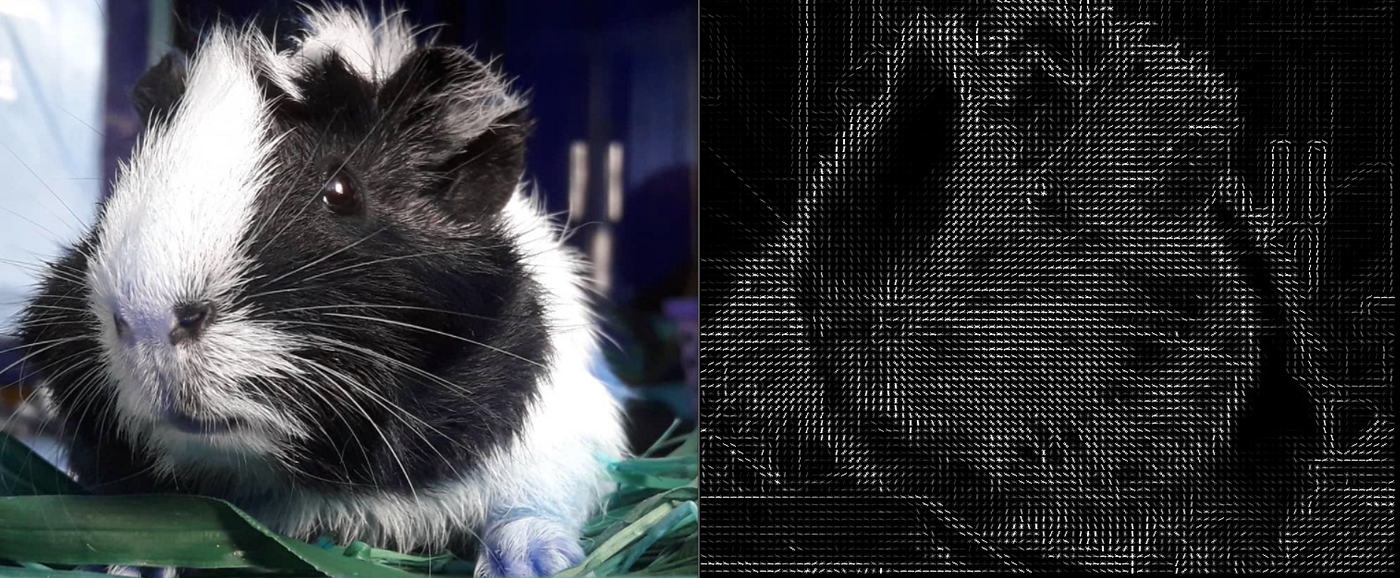Figure (4) - Left Image: Original Image, Right Image: HOG Image created by the author
• Gamma/Color Normalization (Optional)
• Spatial/Orientation Binning (Dividing the image into cells).
• Block Normalization.
• Get the HOG Feature Vector.

Gamma Normalization is a power-law transformation which is also called gamma correction. It is used to adjust illuminance or color intensities. In the paper, the author experimented with different color spaces including gray-scale, RGB and LAB. RGB and LAB color spaces give similar results. However, these variances in illuminance do not have many effects on the HOG descriptor when we apply block normalization. As this is a minor step, you can skip this step or test in your free time to see the effect of changes. You can also use square root normalization and variance normalization. Here, I will show how to make gamma correction.

Gamma Correction equation:

`S = C.R^(gamma)To encode gamma, gamma= 1/gammaTo decode gamma, gamma= gammaC = color intensityR = (Image_input)/C`

In order to detect changes in light intensity, it is required to compute gradients of an image. Either first-order derivative or Sobel mask can be used to proceed. In the research paper, various 1-D point derivatives and masks were used to compare the results, and they found out that a simple 1-D [-1,0,1] mask works best. For color images, you have to compute gradient differences of all channels and pick the largest norm.

The horizontal gradient detects horizontal edges while the vertical gradient detects upright position ones. Using the colored image, the gradient values are sharper and finer than the gray-scale one. These values are not actual values since we are going to use them for building a histogram. We have to take the square root of both X and Y magnitudes. The image shown below is a result of taking the square root of both horizontal and vertical gradient magnitudes. At the same time, you can calculate magnitudes for orientation by using the tangent inverse of Y values and X values (see in figure-4)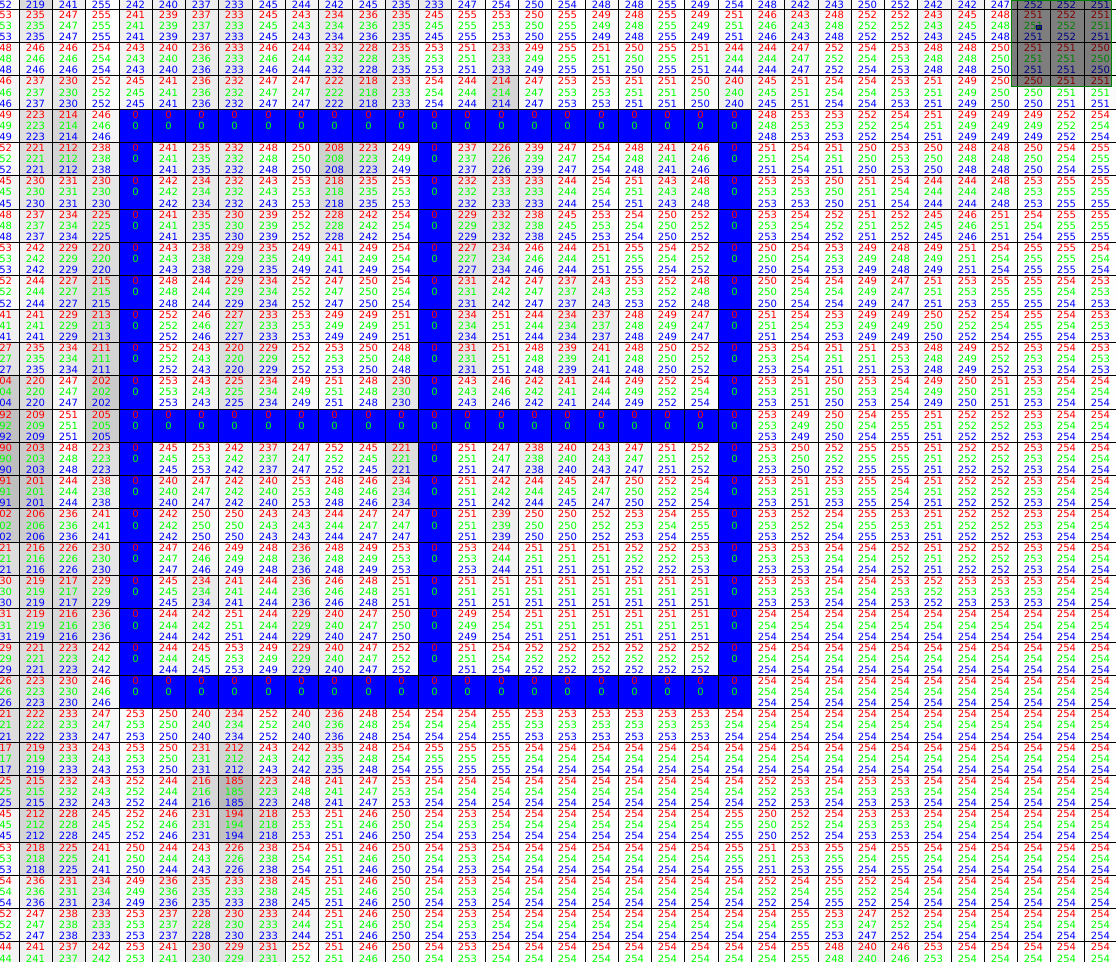Figure (6) - Each rectangle has 2x2 block and 8 x 8 in each grid

The image is scanned using sliding windows technique. If an image has 512 x 512 dimension, 63 x 63 blocks with stride one will be obtained. In each block cell, there are 2 x 2 grid having 8 x 8 pixels values in each. Therefore, gradients and orientations are 8 x 8. Each cell has 8 x 8 x 2 = 128 values.

You can use your customized values for the number of grid cells and blocks and even orientation bins. The default value is 8 x 8 cells with 4 grids per block. From each cell, histograms are drawn with gradient magnitudes and orientations. But, the author stated that increasing the number of orientation bins up to 9 can improve performance. You can also use ‘signed’ (0–180) or ‘unsigned’ (0–360) degree values for your histogram.

For human detection, 3 x 3 cells blocks of 6 x 6 pixel cells performs best. You can see comparisons of different cells blocks and pixels size below.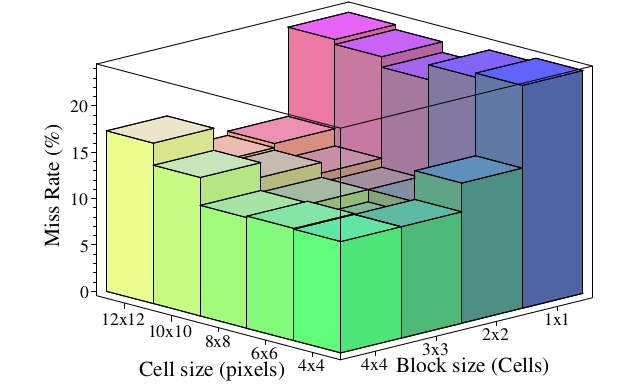Figure (7) - Showing miss rate results of different hyperparameters: block size and cell size [This photo is taken from the paper of Histogram of Oriented Gradient for Human detection Figure no.]

Below code is for calculating histogram.

Step 4: Block Normalization

Block Normalization is crucial to maintain gradient values as these values can vary slightly with illuminance according to background and foreground contrast. To overcome this problem, we can normalize cells. In such cases, we can use block normalization. Block normalization for each 2 x 2 grid cells is simple and straight forward. You can use four different normalization schemes. The researcher found out that L2-Hys, L2-norm and L1-sqrt perform equally and L1-norm reduces the performance by 5 %.

Step 5: Get the HOG feature Vector

After block normalization, the only thing that you will have to perform is to get the feature vector. If we use 9 orientation bin, you have 9 feature vector from each 8x8 grid. For each block of 4 grids, you have 9 x 4 = 36 feature vectors. This means that each 16 x 16 block have 36 x 1 vector.

The number of block in 512 x 512 image is 63x 63, so our final feature vector is 63 x 63 x 36 = 142884 dimensional vector. When you pass an image without HOG descriptor, you will have 512 x 512 x 3 =786432 features. Therefore, total number of HOG features is always smaller than a colored image features. To construct hog feature vector and visualization for histogram is cumbersome and required many steps. If you are very eager to know detail implementations of code in python, see Hog feature in scikit-image documentation.

Reference:

1. “Histograms of Oriented Gradients for Human Detection”. Retrieved 11 May 2021, from http://lear.inrialpes.fr/people/triggs/pubs/Dalal-cvpr05.pdf
2. LSI Design Contest ,from LSI Design Contest. (2021). Retrieved 11 May 2021, from http://www.lsi-contest.com/2017/shiyou_3-1e.html
3. Histogram of Oriented Gradients — skimage v0.18.0 docs. (2021). Retrieved 11 May 2021, from https://scikit-image.org/docs/stable/auto_examples/features_detection/plot_hog.html
4. Wikipedia contributors. (2021, February 1). Histogram of oriented gradients. In Wikipedia, The Free Encyclopedia. Retrieved 04:44, May 11, 2021, from https://en.wikipedia.org/w/index.php?title=Histogram_of_oriented_gradients&oldid=1004270806

Engineering student from Myanmar keen on learning computer vision and AI

## More from Wai Yan Kyaw

Engineering student from Myanmar keen on learning computer vision and AI